# Analysis of the Movement Characteristics of Corona Winds during Needle-Plate Discharge

Analysis of the Movement Characteristics of Corona Winds during Needle-Plate Discharge

Jiewen Deng Hongwei Qu Jianqing Lin Guoxin Yu Qin Deng*

School of Energy Power and Mechanical Engineering, North China Electric Power University, Beijing 102206, China

School of Energy and Power Engineering, Northeast Dianli University, Jilin 132012, China

Nuclear Power Engineering Co., Ltd, Shenzhen 518027, China

Beijing green energy technology Co., Ltd,Beijing 102206, China

Corresponding Author Email:
dengqin1818@163.com
Page:
574-580
|
DOI:
https://doi.org/10.18280/ijht.340404
|
Accepted:
|
Published:
31 December 2016
| Citation

OPEN ACCESS

Abstract:

In order to study corona wind flow during the negative corona discharge of needle plates, a total-ion numerical model of corona winds was established based on a constant-coefficient partial differential equation. By coupling the electrostatic field Poisson equation and the fluid control equation, we analyzed the electro hydrodynamics of needle-plate coronas. At the respective voltages of -9 kV and -16 kV, an experiment was conducted on the velocities of the corona wind flows generated from corona electrodes of three different curvature radii. The corresponding results show that the corona wind’s low velocity at the needle point (the site of the corona) increased rapidly along the axial direction, leveled off at a certain value, and then dropped down. The larger the potential difference between plates, the faster the corona wind; the smaller the radius of curvature of the corona, the faster the corona wind to be generated around the pole tip.

Keywords:

Corona wind, Partial differential equation, Electro hydrodynamics, Air ionization, High voltage discharge.

1. Introduction

Electrostatic precipitator (ESP)  is commonly used in removing charged particles by carrying them towards the plate for absorption under the action of a high-voltage electrostatic field. The key to dust removal performance improvement lies in removing 0.3-1.0 μm particles in a more efficient manner. However, when drifting towards the dust collector plate, particles of this size range can be greatly affected by the flow field near electrode plate which is ruled by the electro fluid formed during needle-plate discharge. Actual operation is frequently accompanied by problems such as dust re-entrainment and cathode line swell [2-4], which is closely linked to the flow field distribution in the vicinity of the electrode plate.

The corona phenomenon in electrostatic precipitator results from self-maintained discharge [5-8] in a very uneven electric field that is formed between plates during the electric discharge around the upper needle end of cathode lines. Due to the formation and quick motion of massive charged ions under the action of the electric field force, their collision with particles in the air triggers an air jet and further disturbs nearby gases, and thus the corona wind forms with the occurrence of a corona . According to many researchers, it is the corona wind generated by the corona in the electrostatic precipitator that mainly propels particles towards the plate side, which has a great impact on the dust removal performance. To overcome this problem, some studies have been undertaken on measurement of corona winds, but the findings differ greatly from each other. For example, Hinds indicated that the speed of corona winds exceeded 75 m/s in the corona region .

Current experimental systems applied to measuring corona wind velocity are complicated and costly, with tracer particles as the medium in most cases . By gauging the speed of tracer particles moving in the air, the corona wind velocity can be calculated and falls within the range of 1-10 m/s [12-15].

In terms of experimentation, Zeng Yuxuan et al.  studied the relationship between input power, electrode spacing and ionic wind velocity by using a two-dimensional laser particle imaging velocimetry technique. With the semi-empirical formula, Ling and Lin  obtained the mean speed (1.162 m/s) of the ionic corona wind between a circular corona electrode and an earth plate. Gong Tao  employed a multi-pin-net structure to have stable electricity discharged. The product of steady-velocity corona winds resulted in an ionic wind of certain speed. Wang Wei  studied the characteristics of thrust of corona winds generated by using the same structure.  Yang Lanjun et al.  applied PIV to the measurement of ionic winds of needle-plate electrodes, whose results resembled the measurement data obtained through laser Doppler vibrometry.

In terms of numerical simulation, Li Qing et al.  used the numerical simulation method to analyze the features of corona wind during wire-plate discharge, and verified their findings in an experiment. Dong Sik Cho  conducted numerical analysis on the influence of charged ions in the dielectric fluid on liquid flow, and proposed a mechanism for the movement of a bidirectional electric water pump. Quentin Lancereau  revealed the connection between the electric field and the flow field by putting forward a model of the electrofluid dynamic field generated in an on-line/cylindrical axisymmetric electrostatic precipitator under laminar flow. As described in the above literature, the mathematical model is generally simplified in various degrees by researchers on electrohydrodynamics. The single-ion method is the most frequently used simplification approach, but it involves a rather complex corona process and produces various types of ions. Since the single ion model ignores the corona process, the method does not apply to corona wind calculation.

In this paper, a numerical simulation was conducted on the generation of corona winds on the foundation of a total-ion discharge model, with the establishment of a COMSOL-based numerical model of electro hydrodynamics. On this basis, constant-coefficient partial differential equations were used to describe the internal relationship between spatial charges, electric field and flow field during the formation of corona winds.

2. Boundary Conditions and Mathematica-L Model

## 1.png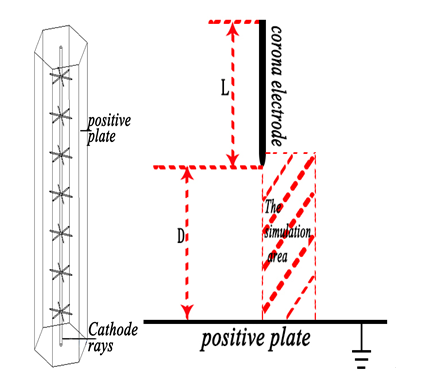Figure 1. Schematic diagram of anode and cathode discharge in ESP

In this paper, the geometrical structure of ESP is simplified as a two-dimensional needle-plate, and the solution area is reduced to a two-dimensional axisymmetric needle-plate structure with the center line of the corona pole as the axis. The increased distance between electrodes raises demands for large-value onset coronas. For the experiment’s sake, we let the grounded plate electrode be a positive plate with a radius of 10 mm, whose spacing from the corona electrode was designated as 10.5 mm. Solutions were found in the finite element [24,25]based COMSOL Multiphysics software. The initial flow velocity was 0, and the coronas generated from the corona electrode were -9 kV and-16 kV, as shown in Figure 1.

The products of corona discharge included electrons, negative ions, positive ions , and neutral ions. For this complex physical process, most researchers sacrifice the accuracy of calculation for the reduction in computational load by using the single-ion method. In this paper, the total-ion mathematical model was employed to describe corona wind .

The flow control equation:

$\rho_{a i r}\left(\frac{\partial v}{\partial t}+(v \bullet \nabla) v\right)=-\nabla p+\mu \Delta v+f$(1)

where: m is the air viscosity coefficient, f is the body force exerted on the flow.

Poisson equation of electrostatic field :

$E=-\nabla V$(2)

$\nabla^{2} V=-\frac{\rho}{\varepsilon_{0}}=-\frac{e\left(n_{p}-n_{n}-n_{e}\right)}{\varepsilon_{0}}$(3)

where: E is the space vector of the electric field, V is the potential of a spatial position (x, y, z), ρ is the bulk density of charge volume at the spatial position (x, y, z), ε0 is the vacuum dielectric constant, and ne ,npand nn represent the ion concentrations of electrons, positive ions and negative ions, respectively.

Ion diffusion equation:where:

$\frac{\partial n_{e}}{\partial t}+\nabla \bullet\left(-\mu_{e} n_{e} \nabla V+n_{e} v-D_{e} \nabla n_{e}\right)=(\alpha-\eta) v_{e} n_{e}$(4)

$\frac{\partial n_{p}}{\partial t}+\nabla \bullet\left(-\mu_{p} n_{p} \nabla V+n_{p} v-D_{p} \nabla n_{p}\right)=-\alpha v_{e} n_{e}$(5)

$\frac{\partial n_{n}}{\partial t}+\nabla \bullet\left(-\mu_{n} n_{n} \nabla V+n_{n} v-D_{n} \nabla n_{n}\right)=\eta v_{e} n_{e}$(6)

$f=\rho_{e} E-\frac{1}{2} E^{2} \nabla \varepsilon+\frac{1}{2} \nabla\left(E^{2} \rho_{\text {air}} \frac{\partial \varepsilon}{\partial \rho_{\text {air}}}\right)$(7)

in which De, Dp and Dn denote the diffusion coefficients of electrons, positive ions and negative ions, respectively; mi is the mobility speed of each ion; α represents the impact-ionization coefficient; η is the adhesion coefficient; ve is the electron drift velocity; v is the flow velocity; f is the vector of electric body force and ρair  is the air density.

3. Results and Discussion

3.1 Electric field distribution

Figure 2 shows the electric field strength of sample points on the edge of the corona pole during the discharge process at different voltages and radiuses of curvature. According to the calculation results, points approaching the tip of the corona pole had higher electric field strengths because of the electrodes geometric effect. Around the pole tip with a small radius of curvature, there was significant spatial change of potential distribution, which contributed to a larger bulging part of the spatial field strength of cathode lines. Accordingly, the electric field inhomogeneity was remarkable such that it resulted in the occurrence of “tip discharge” or corona. As can be seen from the results, the field strength became larger at positions closer to the discharge tip. When the curvature radii of the corona electrodes were the same, the higher the corona voltage, the higher the field strength; and when the corona voltages were equal, the smaller the curvature radii of the corona electrode, the higher the field strength. High field strength was the predominant factor affecting corona generation. The relationships between the radius of curvature of corona tip and the electric field strength coordinated with the conditions in which the electric corona can occur.

## 2.png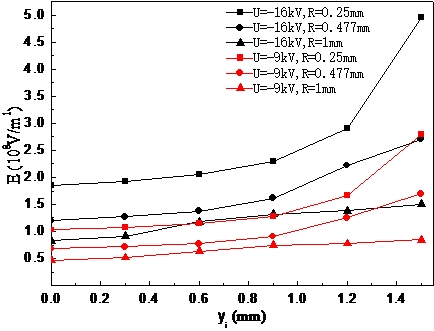Figure 2. The electric field strength at the edge of the corona pole

3.2 Corona wind velocity field distribution

## 3a.png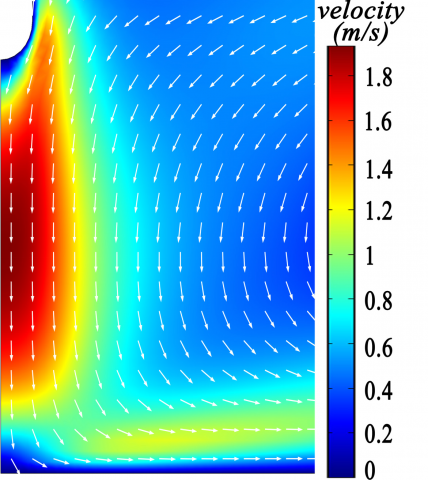At the curvature radius of 1 mm, Corona voltage -9 kV

## 3b.pngAt the curvature radius of 1 mm, Corona voltage -16 kV

## 3c.png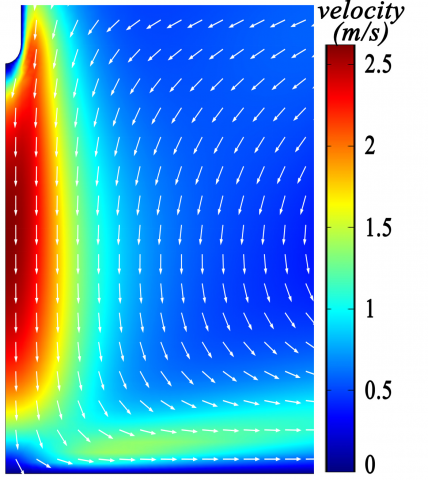At the curvature radius of 0.477 mm, Corona voltage -9 kV

## 3d.pngAt the curvature radius of 0.477 mm, Corona voltage -16 kV

## 3e.png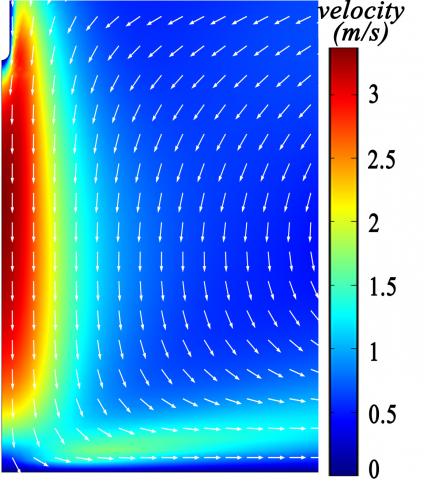At the curvature radius of 0.25 mm, Corona voltage -9 kV

## 3f.pngAt the curvature radius of 0.25 mm, Corona voltage -16 kV

Figure 3. Cloud map of corona wind velocity

Figure 3 shows the distributions of corona wind velocity at a range of curvature radiuses and discharge voltages. The velocity field in the needle plate facing area presents an N shape with higher values in the middle part. The development trends of corona winds at different radii of curvature were similar to each other. By referring to Figure 4, it can be seen that the smaller the absolute value of the voltage, the less the curvature radius responds to corona wind velocity; and the smaller the curvature radius of the needle end, the faster the corona wind becomes. The reason for this phenomenon is that a small curvature radius contributes to the generation of an uneven electric field of high strength much more easily, thus the body force gained by the electro fluid is greater. As a result, the initial force obtained by charged ions generated from corona maximized itself such that the acceleration was relatively large, which further increased the speed of the corona wind. From the velocity vector in the figure, we can see that the corona wind started from the corona pole tip, and moved toward the positive plate until encountering the blocking force against the positive plate. It then decelerated and spread out along the plate surface, leading to nearby gas flow. The trajectory of corona wind became curve-like, which, together with the gas flow, impedes dusts from gathering on the plate, thus reducing the dust removal efficiency.

3.3 Response of corona wind speed to voltage

## 4.png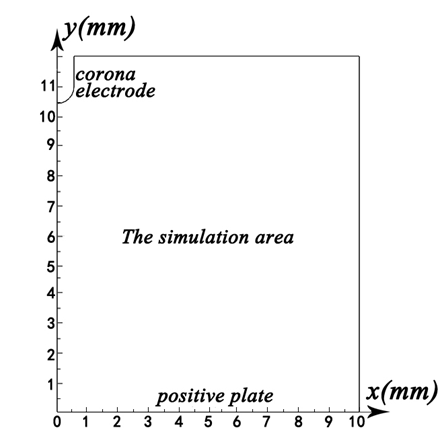Figure 4. Coordinate system of corona wind velocity field

To better analyze the variation of the velocity field near the tip of the corona wind, the coordinate system in Figure 4 was constructed with the direction along the anode plate taken as the X-axis, the direction along the center line of the corona pole as the Y-axis, and the point on the anode plate directly opposed to the center line of the corona pole as the coordinate origin.

Figure 5 shows Y-direction corona wind velocities at different voltages (when X=0, it refers to the central axis of the solution domain). The results show that under the same calculation conditions, areas along the Y direction are mainly divided into the acceleration region, stable region and attenuation region. The farther the wind was from the corona pole, the smaller the velocity. Corona wind formed at the tip of the corona pole. Within a short distance, the velocity increased from 0 to the maximum value, stabilized for a period, and then decreased rapidly. In addition, at different voltages, the larger the absolute value of corona discharge, the faster the corona wind; and the shorter the distance left for acceleration, the larger the axial scope of the steady-speed area. If the potential difference between plates is large, corona wind velocity will increase to the peak value for an instant. This law applies at various curvature radii of needle. By combining equation (2) and (7), it can be seen that the higher the potential difference, the larger the electric flow strength, which intensifies the electric field force and the value of the body force in equation (1). Therefore, the potential gradient distribution is the key to controlling the velocity field distribution in the corona area. As shown in Figure 2, the electric field strength was large near the tip of the corona, which decreased rapidly along the axial direction. Upon the occurrence of corona, charged ions were subject to a strong electric field force (the acceleration field force) such that the corona wind velocity jumped upward from 0. Then, these ions entered an area where the electric field strength was poor. Accordingly, the electric field force exerted on them was weakened. Meanwhile, the corona wind speed rose to the maximum value and then stabilized. When approaching the earth plate, the corona wind decelerated under the blocking force of the baffle plate.

## 5a.png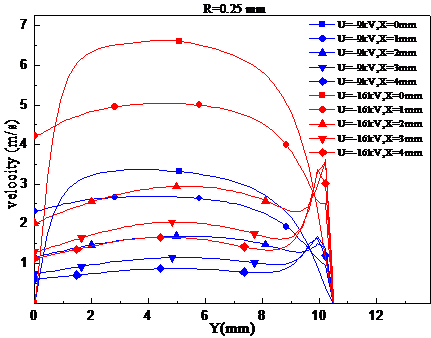## 5b.png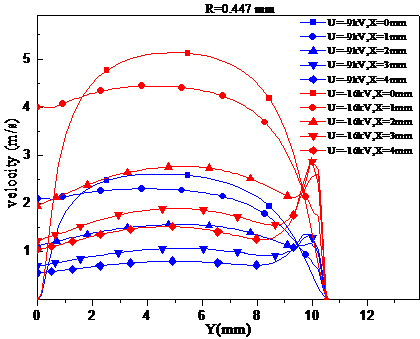## 5c.png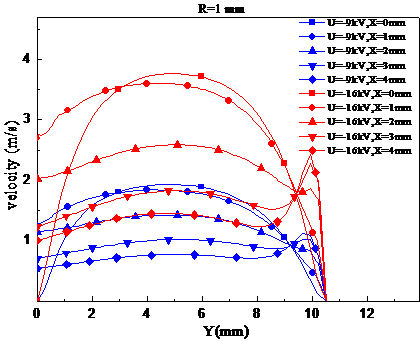Figure 5. Y-direction corona wind velocity at different voltages

Figure 6 shows the change of corona wind velocity in the X direction (when Y = 10.5 mm, it refers to the horizontal position of the discharge tip in the solution domain). It can be seen that the higher the absolute value of the discharge voltage, the larger the corona wind velocity in the horizontal direction, and the easier it is for the wind to attenuate. In addition, the corona wind speed was 0 at the tip of the corona pole, rising quickly to the peak value and then plummeting in the horizontal direction. This phenomenon was commonly seen at different voltages, and the reason is: the flow of corona wind occured in the corona area, whose changes in velocity triggered the velocity change in other positions; the larger the absolute value of the voltage, the larger the field strength, and the greater the electric field force exerted on the electric fluid, which in turn rendered the horizontal diffusion distance of charged particles small. As can be seen from Figure 2, the greater the absolute value of the voltage, the smaller the horizontal width of the high-wind-speed area.

## 6a.png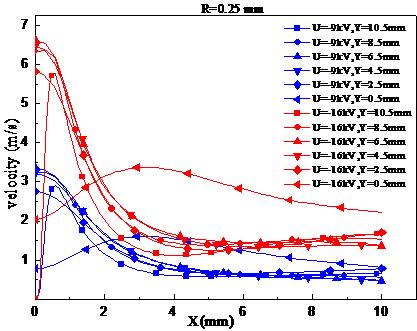## 6b.png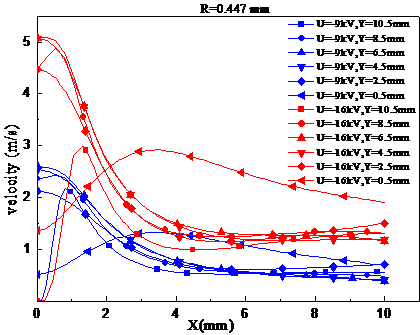## 6c.png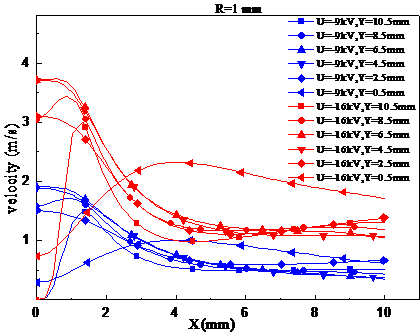Figure 6. Corona wind velocity in X direction at different voltages

3.4 Influence of curvature radius on corona wind velocity

## 7a.png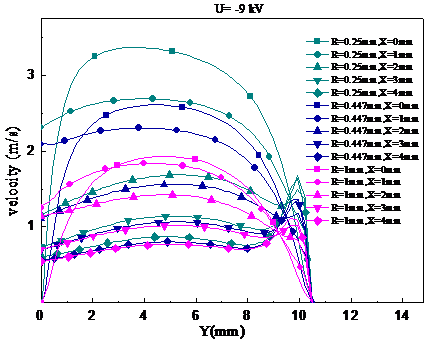## 7b.png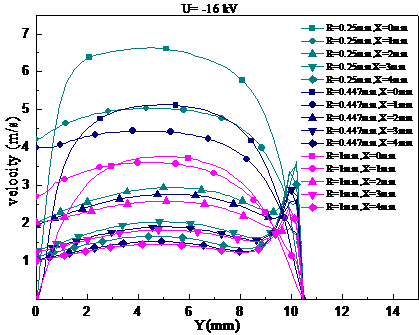Figure 7. Y-direction corona wind velocity at different radii of curvature

Figure 8 shows the X-direction corona wind velocities at different radii of curvature. It was found that the smaller the radius of curvature, the larger the corona wind velocity in the area where the corona is facing the earth plate, and the faster the horizontal speed attenuation, which was reduced to a similar value in the end. In addition, when the curvature radius changed, the horizontal width of the high-wind-speed area also varied. By referring to the speed field in Figure 2, we summarized that the smaller the curvature radius, the narrower the fast-wind-speed area was horizontally. The two-dimensional asymmetrical solution domain used for calculation herein corresponds to the “spindle-like” high-speed corona wind area at the three-dimensional level. This area allowed negatively-charged particles to quickly leave the corona pole, increasing the probability of positively-charged particles to be neutralized. The neutral particles formed drifted towards the plate under the action of the corona wind, during which they could combine with electrons and be absorbed on the dust collector plate resultantly. Therefore, a rational design of corona pole layout can improve the near-corona dust environment by avoiding the clustering of massive dusts in the vicinity of the corona pole.

## 8a.png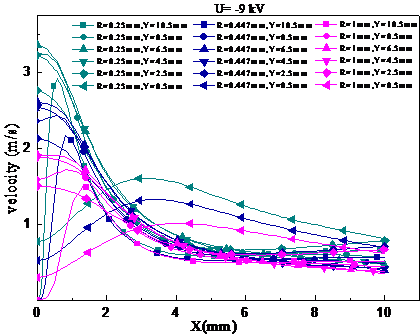## 8b.png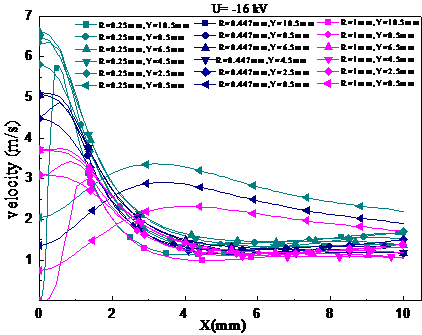Figure 8. X-direction corona wind velocities at different radii of curvature

4. Conclusions

The research in this paper provides theoretical basis for the optimization of the flow field near the corona pole of ESP. The respective corona winds generated at a pair of voltages and three radiuses of curvature were simulated numerically. The main conclusions are:

1) The corona wind mainly forms in the corona area, and the change of the flow field in other locations is caused by the corona wind;

2) Under several corona voltages, all velocities of the corona wind within the corona area are accelerated first and then become stable, followed by reductions. At a fixed spatial position, the higher the absolute voltage value, the higher the corona wind velocity.

3) The change of curvature radius of the corona pole within the corona area contributes little to the stabilized area formed after the attenuation of corona wind. The smaller curvature radius results in a rise in the velocity of the corona wind and the axial width of the high-speed region, as well as the shrinkage of its overall horizontal width.

References

 Yi Chengwu, Liu Shiwen, Shi Xuexin, et al., “Experiment study on the collection performance of single-stage double-vortex collecting plate electrostatic precipitator,” High Voltage Engineering, vol. 42, no. 8, pp. 2651-2658, 2016.

 Li Xin, “The major causes and countermeasures that Influence the Performance parameters of electrical precipitator,” Northeastern University, 2010.

 Pan Xiaohui, Cui Lin, Ma Chunyuan, “Influence of water film on the distribution and motion characteristics of fine particles in electrostatic precipitator,” Proceedings of the CSEE. vol. 36, no. 16, pp. 4333-4342, 2016.

 Huang Bin, Yao Qiang, Li Shuiqing, et al., “Progress in technology of electrostatic enhancement for removal of PM2.5,” Power System Engineering, vol. 19, no. 6, pp. 44-46, 2003.

 Liu Hao, Liu Shanghe, Cao Hefei, et al., “Experimental simulation system of corona discharges under high-altitude, low-pressure and low-temperature conditions,” High Voltage Engineering, vol. 41, no. 2, pp. 578-583, 2015.

 Wang Liming, WAN Shuwei, Bian Xingming, et al., “Uitraviolet characteristics of negative dc corona discharge in extremely non-uniform electric field,” High Voltage Engineering, vol. 40, no. 6, pp. 1614-1622, 2014.

 Yan Keping, Li Shuran, Feng Weiqiang, et al., “Analysis and prospect on key technology of high-voltage discharge for environmental engineering study and application.” High Voltage Engineering, vol. 41, no. 8, pp.2528-2544, 2015.

 He Shoujie, Liu Shumin, Liu Zhiqiang, et al., “Simulation on the characteristics of a slotted hollow cathode discharge.” High Voltage Engineering, vol. 41, no. 2, pp.516-522, 2015.

 Moreau E., “Airflow control by non-thermal plasma actuators,” Journal of Physics D: Applied Physics, vol. 40, no. 3, pp. 605-636, 2007. DOI: 10.1-088/0022-3727/40/3/S01.

 Hinds W. C., Aerosol Technology, New York: John Wiley & Sons Inc, pp. 288-300, 1982.

 Shen Xinjun, Zeng Yuxuan, Zheng Qinzhen, et al., “Measurements of flow field in wire-plate electrostatic precipitator during positive or  negative corona discharge using PIV Method,” High Voltage Engineering, vol. 10, no. 9, pp. 2757-2763, 2014 .

 Nakamura H., Ohyama R., “An image analysis of positive ionic wind velocity under the DC corona discharge in needle-cylinder electrode system,” IEEE Conference on Electrical Insulation and Dielectric Phenomena, pp. 192-195, 2009. DOI: 1- 0.1109/CEIDP.2009.5377857.

 Moreau E., Touchard  G., “Enhancing the mechanical efficiency of electric wind in corona discharges,” Journal of Electrostatics, vol. 66, no. 8, pp. 39-44, 2008.

 Yuan Junxiang, Qiu wei, Zheng Cheng, et al., “Study on characteristics of ionic wind from atmosphere discharge,” Proceedings of the CSEE, vol. 29, no.13, pp. 110-116, 2009.

 Yuan Junxiang, Yang Lanjun, Zhang Qiaogen, et al., “Ionic wind characteristic of tine-plat affected by electrode parameter from atmosphere discharge,” Transactions of China  Electrotechnical Society, vol. 25, no. 1, pp. 24-29, 2010.

 Zeng Yuxuan. Shen Xinjun, Zhang Yuming, et al., “Experimental study of ionic wind in an electrostatic precipitatior,” Journal of Zhejiang University, vol. 47, no. 12, pp. 2208-2211, 2013.

 Liang W J, Lin T. H., “The characteristics of ionic wind and its effect on electrostatic precipitator,” Aerosol Science and Technology, vol. 20, no. 13, pp. 330-344, 1994.

 Gong Tao, Tang Fei, Wang Xiaohao, “Characteristics of ionicwind excited by multiple needles-net structure,” High Voltage Apparatus, vol. 48, no. 5, pp. 33-36, 2012.

 Wang Wei, Yang Lanjun, Gao Jie, et al., “Experimental study on the thrust and the ratio of thrust to power of multi-points/grid ionic wind exciter,” Acta Phys Sin, vol. 62, no.7, pp. 1-7, 2013.

 Li Qing, Li Haifeng, Sun Xiaorong, et al., “Experimental re-search and numerical simulation of electro-hydrodynamic incorona discharge,” High Voltage Engineering, vol. 36, no. 11, pp. 2739-2744, 2010.

 Yang Lanjun, Wang Wei, Lin Cen, et al., “Experimental and theoretical research progress in ionic wind produced by corona discharge and its application,” High Voltage Engineering, vol. 42, no. 2, pp. 1100-1108, 2016.

 Dong Sik Cho, Sangmo Kang, Yong Kweon Suh, et al., “Development of a bi-directional electrohydrodynamic pump: Parametric study with numerical simulation and flow visualization,” Advances in Mechanical Engineering, vol. 8, no. 6, pp. 1–15, 2016. DOI: 10.1177/1687814016655777.

 Quentin Lancereau, Jean-Maxime Roux, Jean-Luc Achard, et al.,  “Electrohydrodynamic flow regimes in a cylindrical electrostatic precipitator,” IEEE Transactions on Dielectrics and Electrical Insulation, vol. 20, no. 4, pp. 1409-1420, 2013.

 Rehena Nasrin, M. A. Alim, “Thermal performance of nanofluid filled solar flat plate collector,” International Journal of Heat and Technology, vol. 33. no. 2, pp 17-24, 2015. DOI: 10.18280/ijht.330203.

 Yueping Qin, Song Kong, Wei Liu, et al., “Dimensionless analysis of the temperature field of surrounding rock in coalface with a finite volume method,” International Journal of Heat and Technology, vol. 33, no. 3, pp 151-157, 2015. DOI: 10.18280/ijht.330323

 Fei-fei Wu, “Numerical simulation of Trichel pulse charateristics in bar-plate DC negative corona discharge,” Acta Physica Sinica, vol. 11,  pp. 338-347, 2013.

 Zhang Bailing, Wang Yutian, Li Yiwen, et al., “Numerical simulation and experimental study for low-pressure direct-current glow discharge,” High Voltage Engineering, vol. 42, no. 3, pp. 724-730, 2016.